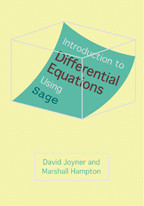Back to Results# Introduction to Differential Equations Using Sage

David Joyner and Marshall Hampton

Publication Date

Differential equations can be taught using Sage as an inventive new approach.

David Joyner and Marshall Hampton's lucid textbook explains differential equations using the free and open-source mathematical software Sage.

Since its release in 2005, Sage has acquired a substantial following among mathematicians, but its first user was Joyner, who is credited with helping famed mathematician William Stein turn the program into a usable and popular choice.

Introduction to Differential Equations Using Sage extends Stein's work by creating a classroom tool that allows both differential equations and...

Differential equations can be taught using Sage as an inventive new approach.

David Joyner and Marshall Hampton's lucid textbook explains differential equations using the free and open-source mathematical software Sage.

Since its release in 2005, Sage has acquired a substantial following among mathematicians, but its first user was Joyner, who is credited with helping famed mathematician William Stein turn the program into a usable and popular choice.

Introduction to Differential Equations Using Sage extends Stein's work by creating a classroom tool that allows both differential equations and Sage to be taught concurrently. It's a creative and forward-thinking approach to math instruction.

Topics include:

• First-Order Differential Equations
• Incorporation of Newtonian Mechanics
• Second-Order Differential Equations
• The Annihilator Method
• Using Linear Algebra with Differential Equations
• Nonlinear Systems
• Partial Differential Equations
• Romeo and Juliet

Reviews

## Reviews

This book, with its many practice examples, would be ideal for those in the first year of a mathematics degree course and for those studying for working in physics or any area requiring a good knowledge of differential equations.

... [Introduction to Differential Equations Using Sage] provides a nice mix of theory and symbolic computation. The pedagogy is excellent and the exercises are models of their kind.

## Book Details

Publication Date
Status
Available
Trim Size
7
x
10
Pages
280
ISBN
9781421406374
Illustration Description
3 halftones, 80 line drawings
Subject

Preface
Acknowledgments
1. First-order differential equations
1.1. Introduction to DEs
1.2. Initial value problems
1.3. Existence of solutions to ODEs
1.3.1. First-order ODEs
1.3.2. Second-order homogeneous

Preface
Acknowledgments
1. First-order differential equations
1.1. Introduction to DEs
1.2. Initial value problems
1.3. Existence of solutions to ODEs
1.3.1. First-order ODEs
1.3.2. Second-order homogeneous ODEs
1.4. First-order ODEs: Separable and linear cases
1.4.1. Separable DEs
1.4.2. Autonomous ODEs
1.4.3. Substitution methods
1.4.4. Linear first-order ODEs
1.5. Isoclines and direction fields
1.6. Numerical solutions: Euler's and improved Euler's method
1.6.1. Euler's method
1.6.2. Improved Euler's method
1.6.3. Euler's method for systems and higher-order DEs
1.7. Numerical solutions II: Runge-Kutta and other methods
1.7.1. Fourth-order Runge-Kutta method
1.8. Newtonian mechanics
1.9. Application to mixing problems
1.10. Application to cooling problems
2. Second-order differential equations
2.1. Linear differential equations
2.1.1. Solving homogeneous constant-coefficient ODEs
2.2. Linear differential equations, revisited
2.3. Linear differential equations, continued
2.4. Undetermined coefficients method
2.4.1. Simple case
2.4.2. Nonsimple case
2.5. Annihilator method
2.6. Variation of parameters
2.6.1. The Leibniz rule
2.6.2. The method
2.7. Applications of DEs: Spring problems
2.7.1. Introduction: Simple harmonic case
2.7.2. Simple harmonic case
2.7.3. Free damped motion
2.7.4. Spring-mass systems with an external force
2.8. Applications to simple LRC circuits
2.9. The power of series method
2.9.1. Part 1
2.9.2. Part 2
2.10. The Laplace transform method
2.10.1. Part 1
2.10.2. Part 2
2.10.3. Part 3
3. Matrix theory and systems of DEs
3.1. Quick survey of linear algebra
3.1.1. Matrix arithmetic
3.2. Row reduction and solving systems of equations
3.2.1. The Gauss elimination game
3.2.2. Solving systems using inverses
3.2.3. Computing inverses using row reduction
3.2.4. Solving higher-dimensional linear systems
3.2.5. Determinants
3.2.6. Elementary matrices and computation of determinants
3.2.7. Vector spaces
3.2.8. Bases, dimension, linear independence, and span
3.3. Application: Solving systems of DEs
3.3.1. Modeling battles using Lanchester's equations
3.3.2. Romeo and Juliet
3.3.3. Electrical networks using Laplace transforms
3.4. Eigenvalue method for systems of DEs
3.4.1. Motivation
3.4.2. Computing eigenvalues
3.4.3. The eigenvalue method
3.4.4. Examples of the eigenvalue method
3.5. Introduction to variation of parameters for systems
3.5.1. Motivation
3.5.2. The method
3.6. Nonlinear systems
3.6.1. Linearizing near equilibria
3.6.2. The nonlinear pendulum
3.6.3. The Lorenz equations
3.6.4. Zombies attack
4. Introduction to partial differential equations
4.1. Introduction to separation of variables
4.1.1. The transport or advection equation
4.1.2. The heat equation
4.2. The method of superposition
4.3. Fourier, sine, and cosine series
4.3.1. Brief history
4.3.2. Motivation
4.3.3. Definitions
4.4. The heat equation
4.4.1. Method for zero ends
4.4.2. Method for insulated ends
4.4.3. Explanation via separation of variables
4.5. The wave equation in one dimension
4.5.1. Methods
4.6. The Schrodinger equation
4.6.1. Method
Bibliography
Index

Author Bios
Featured Contributor

## David Joyner

David Joyner is a professor in the Mathematics Department at the U.S. Naval Academy. He is the author of Adventures in Group Theory: Rubik's Cube, Merlin's Machine, and Other Mathematical Toys, also published by Johns Hopkins.
Featured Contributor